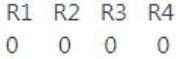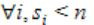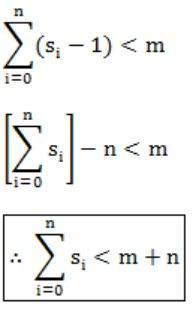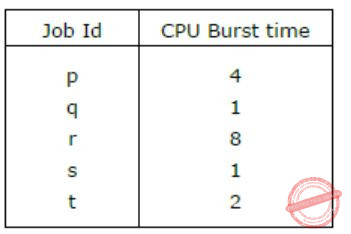Question 1

Consider the following snapshot of a system running n concurrent processes. Process i is holding Xi instances of a resource R, 1 ≤ i ≤ n. Assume that all instances of R are currently in use. Further, for all i, process i can place a request for at most Yi additional instances of R while holding the Xi instances it already has. Of the n processes, there are exactly two processes p and q such that Yp = Yq = 0. Which one of the following conditions guarantees that no other process apart from p and q can complete execution?

 A Min (Xp, Xq) ≥ Min {Yk | 1 ≤ k ≤ n, k ≠ p, k ≠ q} B Xp + Xq < Max {Yk | 1 ≤ k ≤ n, k ≠ p, k ≠ q} C Min (Xp, Xq) ≤ Max {Yk | 1 ≤ k ≤ n, k ≠ p, k ≠ q} D Xp + Xq < Min {Yk | 1 ≤ k ≤ n, k ≠ p, k ≠ q}
Question 1 Explanation:
{P1, P2, ..., Pn}
Pi holds Xi instances.
Pi can request additional Yi instances.
Given two process p & q such that their additional requests are zero.
Yp = Yq = 0
{Yk | 1 ≤ k ≤ n, k ≠ p, k ≠ q} means that out of 'n' processes, we are left with (n-2) process (except p&q), i.e., Yk indicates additional request of all the processes (n-2) except p & q.
For p & q to complete first, accordingly
Xp + Xq < Min {Yk}
Option D is correct.
There are exactly two process p and q which do not need any additional instances of resources.
So, p and q will complete their execution and will release Xp and Xq instances of resources.
Now to guarantee that no other process apart from p and q can complete execution, the no. of instances of resources available must be less than the minimum no. of instances of resources required by any other process, i.e.,
Xp + Xq < Min {Yk | 1 ≤ k ≤ n, k ≠ p, k ≠ q}.
 Question 2

Consider a system with 3 processes that share 4 instances of the same resource type. Each process can request a maximum of K instances. Resource instances can be requested and released only one at a time. The largest value of K that will always avoid deadlock is _________.

 A 2 B 3 C 4 D 5
Question 2 Explanation:
No. of process = 3
No. of resources = 4
Let’s distribute each process one less than maximum demands i.e., (k-1) resources.
So, for three processes, 3(k – 1) resources.
For deadlock avoidance provide an additional resource to any one of the process.
∴ Total resources required to avoid deadlock in any case is 3(k – 1) + 1 = 3k – 2
Now this 3k – 2 should be less than equal to available no. of resources, i.e.,
3k – 2 ≤ 4
k ≤ 2
So maximum value of k = 2
 Question 3

In a system, there are three types of resources: E, F and G. Four processes P0, P1, P2 and P3 execute concurrently. At the outset, the processes have declared their maximum resource requirements using a matrix named Max as given below. For example, Max[P2, F] is the maximum number of instances of F that P2 would require. The number of instances of the resources allocated to the various processes at any given state is given by a matrix named Allocation.

Consider a state of the system with the Allocation matrix as shown below, and in which 3 instances of E and 3 instances of F are the only resources available.From the perspective of deadlock avoidance, which one of the following is true?

 A The system is in safe state. B The system is not in safe state, but would be safe if one more instance of E were available. C The system is not in safe state, but would be safe if one more instance of F were available. D The system is not in safe state, but would be safe if one more instance of G were available.
Question 3 Explanation:
〈E, F, G〉 = 〈3, 3, 0〉Safe sequence:
〈P0, P2, P1, P3
P0: P0 can be allotted 〈3,3,0〉.
After completion Available = 〈4,3,1〉
P2: P2 can be allotted 〈0,3,0〉.
After completion: Available = 〈5,3,4〉
P1: P1 can be allotted 〈1,0,2〉.
After completion: Available = 〈6,4,6〉
P3: P3 can be allotted 〈3,4,1〉.
After completion: Available = 〈8,4,6〉
 Question 4

A system shares 9 tape drives. The current allocation and maximum requirement of tape drives for three processes are shown below:Which of the following best describe current state of the system?

Operating-Systems       deadlock       GATE 2017 [Set-2]       Video-Explanation
Question 4 Explanation:Available: (9 - (3 + 1 + 3)) = 2, P3 can be satisfied.
New available = 3 + 2 = 5
Now, P2 can be satisfied.
New available: 5 + 1 = 6
Now, P1 can be satisfied. Thus safe sequence: P3 → P2 → P1
 Question 5

A system has 6 identical resources and N processes competing for them. Each process can request atmost 2 resources. Which one of the following values of N could lead to a deadlock?

 A 1 B 2 C 3 D 6
Question 5 Explanation:
Let's assume that each process request 2 resources each. Now there are total 6 identical resources available. Give 1 resource to every processes then there will be deadlock because now each process will wait for another resource which is not available, so there will be deadlock. Since there are total 6 resources so for deadlock to be possible there should be 6 processes available. Hence, the value of N is 6.
 Question 6

Consider the following policies for preventing deadlock in a system with mutually exclusive resources.

I. Processes should acquire all their resources at the beginning of execution. If any resource is not available, all resources acquired so far are released.
II. The resources are numbered uniquely, and processes are allowed to request for resources only in increasing resource numbers.
III. The resources are numbered uniquely, and processes are allowed to request for resources only in decreasing resource numbers.
IV. The resources are numbered uniquely. A process is allowed to request only for a resource with resource number larger than its currently held resources.

Which of the above policies can be used for preventing deadlock?

 A Any one of I and III but not II or IV B Any one of I, III, and IV but not II C Any one of II and III but not I or IV D Any one of I, II, III, and IV
Question 6 Explanation:
 Question 7

A system contains three programs and each requires three tape units for its operation. The minimum number of tape units which the system must have such that deadlocks never arise is _________.

 A 7 B 8 C 9 D 10
Question 7 Explanation:
(3*2 tape units) + 1 tape unit = 7
 Question 8

A system has n resources R0,...,Rn-1,and k processes P0,....Pk-1.The implementation of the resource request logic of each process Pi is as follows:

```if (i % 2 == 0) {
if (i < n) request Ri
if (i+2 < n) request Ri+2
}
else {
if (i < n) request Rn-i
if (i+2 < n) request Rn-i-2
}```

In which one of the following situations is a deadlock possible?

 A n = 40, k = 26 B n = 21, k = 12 C n = 20, k = 10 D n = 41, k = 19
Question 8 Explanation:
Consider the case where i = 10 & i = 11, n = 21 & k = 12
P10 requests R10 & R11
P11 requests R10 & R8
Hence P10 & P11 inorder in deadlock.
 Question 9

Consider a system with 4 types of resources R1 (3 units), R2 (2 units), R3 (3 units), R4 (2 units). A non-preemptive resource allocation policy is used. At any given instance, a request is not entertained if it cannot be completely satisfied. Three processes P1, P2, P3 request the sources as follows if executed independently.Which one of the following statements is TRUE if all three processes run concurrently starting at time t=0?

 A All processes will finish without any deadlock. B Only P1 and P2 will be in deadlock. C Only P1 and P3 will be in a deadlock. D All three processes will be in deadlock.
Question 9 Explanation:
At t=0,
→ P1 requests 2 units of R2 which is granted.
→ P2 requests 2 units of R3 which is granted.
→ P3 requests 1 units of R4 which is granted.
Now Available resources are,At t=1,
→ P1 requests 1 unit of R3 which is granted because it is available.
Now Available resources are,At t=2,
→ P2 requests 1 unit of R4 which is granted.
→ P3 requests 2 units of R1.
Now Available resources are,At t=3,
→ P1 requests 2 units of R1 which cannot be granted, and will wait for other processes to release.
Available resources are,At t=4,
→ P2 requests 1 unit of R1, which is granted.
Available resources areAt t=5,
→ P3 releases 2 units of R1.
Now Available resources are,→ Now P1 is waiting for R1, so now P1 will be granted 2 units of R1.
Now Available resources are,→ Now P1 releases 1 unit of R2 and 1 unit of R1.
Now Available resources areAt t=6,
→ Now P2 releases 1 unit of R3.
Now available resources are,At t=7,
→ P3 requests 1 unit of R2, which is granted.
→ P1 releases 1 unit of R3.
Now Available resources are,At t=8,
→ P2 fnishes and releases remaining resources. So now Available resources,→ P3 requests 1 unit of R3 which is granted.
Now Available resources are,→ P1 requests 2 units of R4 which cannot be granted, so it will wait for other process to release them.
At t=9,
→ P3 finishes, and releases rest of the resources.
Now Available resources are→ Now, P1 can be granted with resources 2 units of R4 for which it was waiting for.
Now Available resources are,At t=10,
→ P1 finishes its execution.
So, finally we can conclude that all processes will finish without any deadlock.
 Question 10

Which of the following is NOT true of deadlock prevention and deadlock avoidance schemes?

 A In deadlock prevention, the request for resources is always granted if the resulting state is safe B In deadlock avoidance, the request for resources is always granted if the result state is safe C Deadlock avoidance is less restrictive than deadlock prevention D Deadlock avoidance requires knowledge of resource requirements a priori
Question 10 Explanation:
Deadlock prevention scheme handles deadlock by making sure that one of the four necessary conditions don't occur. So, it may be the case that a resource request might be rejected even if the resulting state is safe. Hence, option (A) is false.
 Question 11

Consider the following snapshot of a system running n processes. Process i is holding xi instances of a resource R, 1≤i≤n. Currently, all instances of R are occupied. Further, for all i, process i has placed a request for an additional yi instances while holding the xi instances it already has. There are exactly two processes p and q such that yp = yq = 0. Which one of the following can serve as a necessary condition to guarantee that the system is not approaching a deadlock?

 A min (xp, xq) < maxk≠p,qyk B xp + xq ≥ mink≠p,qyk C max (xp, xq) > 1 D min (xp, xq) > 1
Question 11 Explanation:
Deadlock refers stops the execution of process due to non-availability of resources.
→ When two (or) more processes waiting for another process to release the resources.
→ P and Q can execute if they have sufficient resources, they don’t wait for extra resources (i.e., Xp+ Xq) required.
→ Option B can satisfies the corresponding equation i.e., Xp+ Xq >= min(Yk) where k != p and k != q.
Here we have sufficient resources.
 Question 12

Suppose n processes, P1, …., Pn share m identical resource units, which can be reserved and released one at a time. The maximum resource requirement of process Pi is si, where si>0. Which one of the following is a sufficient condition for ensuring that deadlock does not occur?

 ABCDQuestion 12 Explanation:
In the extreme situation, we have si - 1 resources and we require one more resource.
If the deadlock will never occur in the corresponding process then the following condition be true.Question 13

Which of the following is NOT a valid deadlock prevention scheme?

 A Release all resources before requesting a new resource B Number the resources uniquely and never request a lower numbered resource than the last one requested C Never request a resource after releasing any resource D Request and all required resources be allocated before execution
Question 13 Explanation:
Given statement is wrong. We can request a resource after releasing any resource.
 Question 14

A  computer  has  six  tape  drives,  with  n  processes  competing  for  them.  Each process may need two drives. What is the maximum value of n for the system to be deadlock free?

 A 6 B 5 C 4 D 3
Question 14 Explanation:
Each process needs 2 drives. So for deadlock just give each process one drive. So total 6 process can be given 1 drive each and can cause deadlock. So to break deadlock just reduce 1 process.
So maximum no. of process for the system to be deadlock free is 5.
 Question 15

An operating system contains 3 user processes each requiring 2 units of resource R. The minimum number of units of r such that no deadlocks will ever arise is

 A 3 B 5 C 4 D 6
Question 15 Explanation:
For the system to cause deadlock give each process 1 resource less than they require. Since in this case they require 2 resource each, so just give them 1 resource each. So if at max if 3 resource will be available then there can be deadlock. So by adding one more resource deadlock will never occur. So in total minimum 4 resources are required so that deadlock will never occur.
 Question 16

A solution to the Dining Philosophers Problem which avoids deadlock is

 A ensure that all philosophers pick up the left fork before the right fork B ensure that all philosophers pick up the right fork before the left fork C ensure that one particular philosopher picks up the left fork before the right fork, and that all other philosophers pick up the right fork before the left fork D None of the above
Question 16 Explanation:
In the Dining philosopher problem, each philosopher needs exactly two chopsticks to eat food but the problem is: each philosopher is going to take one chopstick at a time, which is placed at its right-hand side or at its left-hand side, but remember all should choose in the same manner like if first one chooses in a clockwise manner then each one should choose in clockwise, this type of picking cause, a circular waiting loop because each one is depending on other. This is also called as circular waiting and it leads to deadlock.
To avoid this, atleast one philosopher should choose its first chopstick in different way so that circular loop is not formed.
 Question 17

A computer system uses the Banker’s Algorithm to deal with deadlocks. Its current state is shown in the table below, where P0, P1, P2 are processes, and R0, R1, R2 are resources types.(a) Show that the system can be in this state.
(b) What will the system do on a request by process P0 for one unit of resource type R1?

 A Theory Explanation.
 Question 18

Consider a system having m resources of the same type. These resources are shared by 3 processes A, B and C, which have peak demands of 3, 4 and 6 respectively. For what value of m deadlock will not occur?

 A 7 B 9 C 13, 15 D 13 E 15
Question 18 Explanation:
A requires 3, B-4, C-6;
→ If A have 2, B have 3, C have 5 then deadlock will occur i.e., 2+3+5=10.
→ If we have one extra resource then deadlock will not occur i.e., 10+1=11.
→ If we have equal (or) more than 11 resources then deadlock will never occur.
 Question 19

Assume that the following jobs are to be executed on a single processor systemThe jobs are assumed to have arrived at time 0+ and in the order p, q, r, s, t. Calculate the departure time (completion time) for job p if scheduling is round robin with time slice 1.

 A 4 B 10 C 11 D 12 E None of the above
Question 19 Explanation:
Algorithm: Round robin; TQ: 1p is departure at 11.
 Question 20

A computer system has 6 tape drives, with n process completing for them. Each process may need 3 tape drives. The maximum value of n for which the system is guaranteed to be deadlock free is:

 A 2 B 3 C 4 D 1
Question 20 Explanation:
Lets give 2 tape driver to each process, so that there will be deadlock. So 3 processes will be given two drives each so that there will be deadlock. So to avoid deadlock maximum no. of process should be 1 less than the minimum no. of process that will cause deadlock. So for n=2, the system is guaranteed to be deadlock free.
 Question 21

An operating system implements a policy that requires a process to release all resources before making a request for another resource. Select the TRUE statement from the following:

 A Both starvation and deadlock can occur B Starvation can occur but deadlock cannot occur C Starvation cannot occur but deadlock can occur D Neither starvation nor deadlock can occur